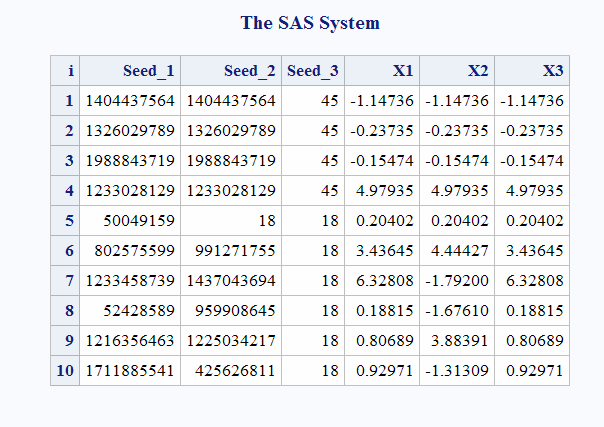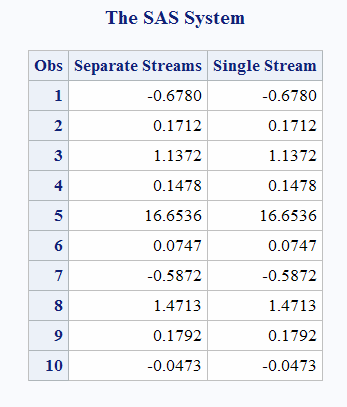# CALL RANCAU Routine

Returns a random variate from a Cauchy distribution.

 Category: Random Number

## Syntax

CALL RANCAU(seed,x);

### Required Arguments

#### seed

is the seed value. A new value for seed is returned each time CALL RANCAU is executed.

 Range seed < 231 - 1 Note If seed ≤ 0, the time of day is used to initialize the seed stream. See Seed Values and Comparison of Seed Values in Random-Number Functions and CALL Routines for more information about seed values

#### x

is a numeric SAS variable. A new value for the random variate x is returned each time CALL RANCAU is executed.

## Details

The CALL RANCAU routine updates seed and returns a variate x that is generated from a Cauchy distribution that has a location parameter of 0 and scale parameter of 1.
By adjusting the seeds, you can force streams of variates to agree or disagree for some or all of the observations in the same, or in subsequent, DATA steps.
An acceptance-rejection procedure applied to RANUNI uniform variates is used. If u and v are independent uniform (−1/2, 1/2) variables and u2+v2 ≤ 1/4, then u/v is a Cauchy variate.
For a discussion and example of an effective use of the random number CALL routines, see Starting, Stopping, and Restarting a Stream.

## Comparisons

The CALL RANCAU routine gives greater control of the seed and random number streams than does the RANCAU function.

## Example

This example uses the CALL RANCAU routine.
```data case;
retain Seed_1 Seed_2 Seed_3 45;
do i=1 to 10;
call rancau(Seed_1,X1);
call rancau(Seed_2,X2);
X3=rancau(Seed_3);
if i=5 then
do;
Seed_2=18;
Seed_3=18;
end;
output;
end;
run;

proc print;
id i;
var Seed_1-Seed_3 X1-X3;
run;```
Output from the CALL RANCAU RoutineThe following is another example of the CALL RANCAU routine:
```data u1(keep=x);
seed = 104;
do i = 1 to 5;
call rancau(seed, X);
output;
end;
call symputx('seed', seed);
run;

data u2(keep=x);
seed = &seed;
do i = 1 to 5;
call rancau(seed, X);
output;
end;
run;

data all;
set u1 u2;
z = rancau(104);
run;

proc print label;
label x = 'Separate Streams' z = 'Single Stream';
run;```
Output from the CALL RANCAU Routine